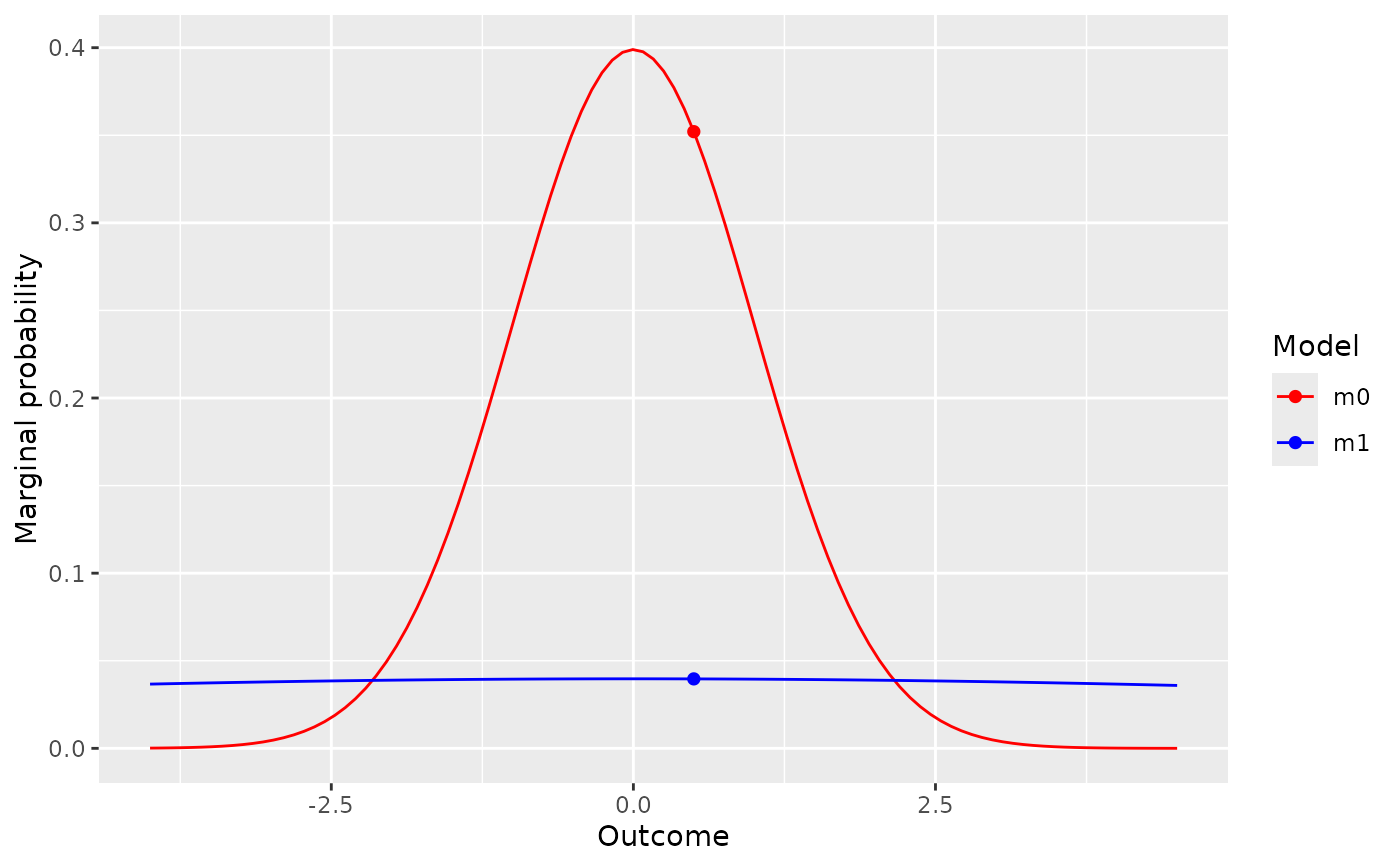Visually compare two models

visual_compare(model1, model2, ratio = FALSE)

## Arguments

model1

a predictive object

model2

a predictive object

ratio

show ratio rather than comparison (default: FALSE)

## Value

A ggplot2 object

## Examples

# define two models
data_model <- likelihood(family = "normal", .5, 1)
h0_mod <- prior(family = "point", point = 0)
h1_mod <- prior(family = "normal", mean = 0, sd = 10)
m0 <- extract_predictions(data_model * h0_mod)
m1 <- extract_predictions(data_model * h1_mod)

# visually compare the model
visual_compare(m0, m1)# plot the ratio of the two model predictions
visual_compare(m0, m1, ratio = TRUE)